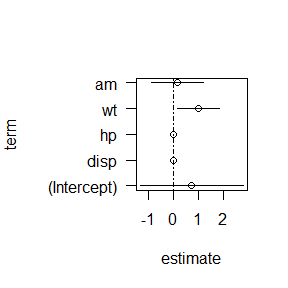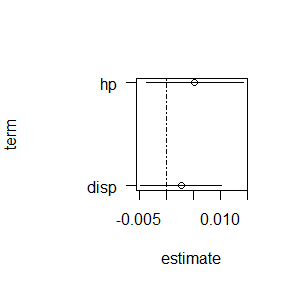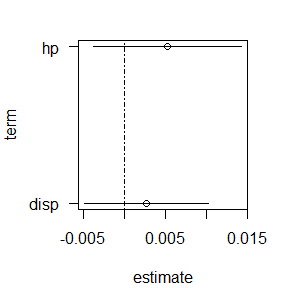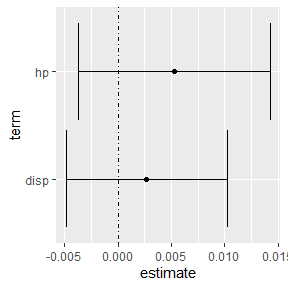# Simultaneous Inference

#### 2022-09-23

The api2lm package makes it easier to perform simultaneous inference for confidence intervals of regression coefficients and the response mean, as well as the prediction intervals for a new response.

Let’s fit a basic basic linear model using the mtcars available in the datasets package. We consider the model fit in the confint.lm function.

fit <- lm(100/mpg ~ disp + hp + wt + am, data = mtcars)

# Confidence for regression coefficients

We construct typical $$t$$-based confidence intervals using the confint function, as shown below. We use the default individual confidence level of 0.95.

confint(fit)
##                    2.5 %      97.5 %
## (Intercept) -0.774822875 2.256118188
## disp        -0.002867999 0.008273849
## hp          -0.001400580 0.011949674
## wt           0.380088737 1.622517536
## am          -0.614677730 0.926307310

The family-wise confidence level is guaranteed to be at least $$1-5(0.05)\geq 0.75$$ based on Boole’s inequality. We can use the Bonferroni correction or the Working-Hotelling procedure (the latter applies to all linear combinations of the regression coefficients) to control the family-wise confidence level. These adjusted intervals are available using the confint_adjust function in the api2lm package. By default, the function makes no adjustments, but indicates the lower bound of the family-wise confidence level.

library(api2lm)
confint_adjust(fit)
##
##
## Family-wise confidence level of at least 0.75
##
##         term          lwr         upr
##  (Intercept) -0.774822875 2.256118188
##         disp -0.002867999 0.008273849
##           hp -0.001400580 0.011949674
##           wt  0.380088737 1.622517536
##           am -0.614677730 0.926307310

We get the same intervals as before, but the print method for the object returned by confint_adjust provides the family-wise confidence level.

To use a Bonferroni adjustment, we set method = "bonferroni" for confint_adjust, as shown below.

(ci_b <- confint_adjust(fit, method = "bonferroni"))
##
##
## Family-wise confidence level of at least 0.95
##
##         term          lwr        upr
##  (Intercept) -1.305763263 2.78705858
##         disp -0.004819755 0.01022561
##           hp -0.003739190 0.01428828
##           wt  0.162448204 1.84015807
##           am -0.884617388 1.19624697

Naturally, these intervals are wider than the unadjusted intervals but control the family-wise confidence level at 0.95.

To construct the Working-Hotelling-adjusted intervals, adjustment, we set method = "wh" for confint_adjust, as shown below.

confint_adjust(fit, method = "wh")
##
##
## Family-wise confidence level of at least 0.95
##
##         term          lwr        upr
##  (Intercept) -1.907955577 3.38925089
##         disp -0.007033435 0.01243929
##           hp -0.006391640 0.01694073
##           wt -0.084399576 2.08700585
##           am -1.190782809 1.50241239

The Working-Hotelling adjusted intervals are even wider than the Bonferroni-adjusted intervals, so the Bonferroni correction is preferred here.

We can easily plot our confidence intervals using plot or autoplot (if the ggplot2 package is available. We plot the Bonferroni-adjusted intervals stored in ci_b using the code below.

plot(ci_b)The intervals for hp and disp are difficult to see using because of their scale relative to the other intervals, so we use the parm function to look at them specifically in the code below.

plot(ci_b, parm = c("hp", "disp"))The intervals are now easier to see. We can use the mar argument to reduce the margin along the y-axis (which is modified internally by default so that all interval labels are shown).

plot(ci_b, parm = c("hp", "disp"), mar = c(4.1, 4.1, 2.1, 2.1))Alternatively, we can use the autoplot function, which makes these adjustments automatically.

library(ggplot2)
autoplot(ci_b, parm = c("hp", "disp"))# Confidence intervals for response mean

Interval procedures, whether for the response mean or new observations, suffer from the same type of multiple comparisons problems that intervals for regression coefficients have.

The predict_adjust function can be used to create adjusted intervals that control the family-wise confidence level for the mean response. The function can be used to produce unadjusted intervals (method = "none"), Bonferroni-adjusted intervals (method = "bonferroni"), and Working-Hotelling-adjusted intervals (method = "wh"). The Working-Hotelling intervals will tend to be narrower the more intervals considered because the Working-Hotelling procedure is valid for ALL linear combinations of the regression coefficients and not only the ones being produced. We produce unadjusted, Bonferroni-adjusted, and Working-Hotelling-adjusted intervals for two combinations of predictors in the code below.

# observations for which to predict the mean response
newdata <- as.data.frame(rbind(
apply(mtcars, 2, mean),
apply(mtcars, 2, median)))
interval = "confidence",
method = "none")
##
##
## Family-wise confidence level of at least 0.9
##
##        fit      lwr      upr
## 1 5.422724 5.177744 5.667704
## 2 5.249334 4.838696 5.659972
# bonferroni-adjusted intervals
interval = "confidence",
method = "bonferroni")
##
##
## Family-wise confidence level of at least 0.95
##
##        fit      lwr      upr
## 1 5.422724 5.139347 5.706100
## 2 5.249334 4.774336 5.724332
# working-hotelling-adjusted intervals
interval = "confidence",
method = "wh")
##
##
## Family-wise confidence level of at least 0.95
##
##        fit      lwr      upr
## 1 5.422724 4.994569 5.850879
## 2 5.249334 4.531657 5.967011

# Prediction intervals for a new response

The predict_adjust function can be used to create adjusted intervals that control the family-wise confidence level for new responses. The function can be used to produce unadjusted intervals (method = "none"), Bonferroni-adjusted intervals (method = "bonferroni"), and Scheffe-adjusted intervals (method = "scheffe").We produce unadjusted, Bonferroni-adjusted, and Scheffe-adjusted predictions intervals for four combinations of predictors in the code below.

# observations for which to predict the mean response
newdata <- as.data.frame(rbind(
apply(mtcars, 2, mean),
apply(mtcars, 2, median),
apply(mtcars, 2, quantile, prob = 0.25),
apply(mtcars, 2, quantile, prob = 0.75)))
interval = "prediction",
method = "none")
##
##
## Family-wise confidence level of at least 0.8
##
##        fit      lwr      upr
## 1 5.422724 4.015419 6.830029
## 2 5.249334 3.803957 6.694711
## 3 4.160836 2.658243 5.663429
## 4 6.341739 4.797332 7.886146
# bonferroni-adjusted intervals
interval = "prediction",
method = "bonferroni")
##
##
## Family-wise confidence level of at least 0.95
##
##        fit      lwr      upr
## 1 5.422724 3.587138 7.258310
## 2 5.249334 3.364089 7.134579
## 3 4.160836 2.200963 6.120709
## 4 6.341739 4.327326 8.356151
# scheffe-adjusted intervals
interval = "prediction",
method = "scheffe")
##
## 4 6.341739 3.855437 8.828040